<%intNum = Request.QueryString("num")%> Photo of Headquarters Company in May 1919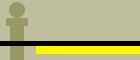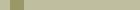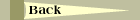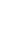<%if intNum = 1 then%>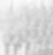<%else%><%end if%> <%if intNum = 2 then%><%else%><%end if%> <%if intNum = 3 then%><%else%><%end if%> <%if intNum = 4 then%><%else%><%end if%> <%if intNum = 5 then%><%else%><%end if%> <%if intNum = 6 then%><%else%><%end if%> <%if intNum = 7 then%><%else%><%end if%> <%if intNum = 8 then%><%else%><%end if%>
<%if intNum = 0 then%>

Click photo section for larger view.

Note company mascot, "Minnie," sitting atop the base drum in the fourth photo from the left. <%elseif intNum = 1 then%>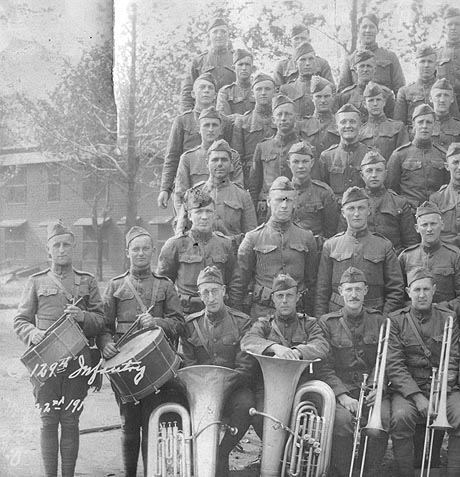<%elseif intNum = 2 then%>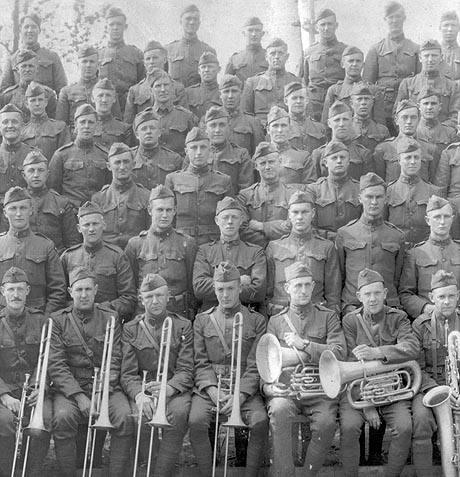<%elseif intNum = 3 then%>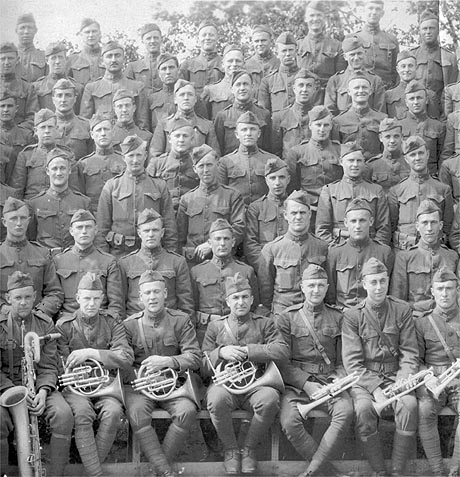<%elseif intNum = 4 then%>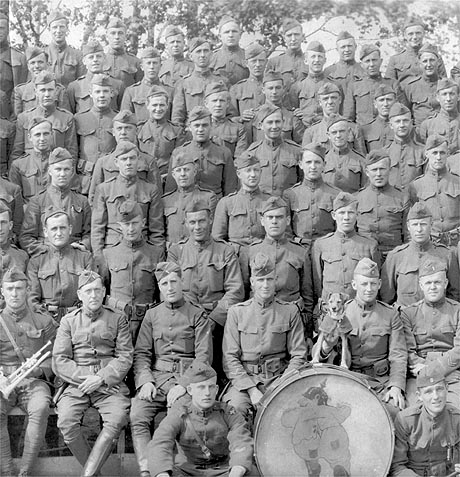<%elseif intNum = 5 then%>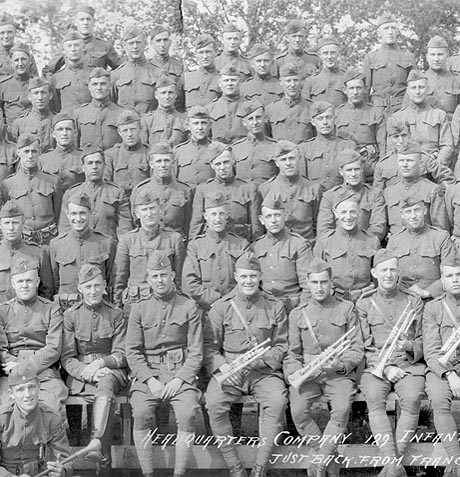<%elseif intNum = 6 then%>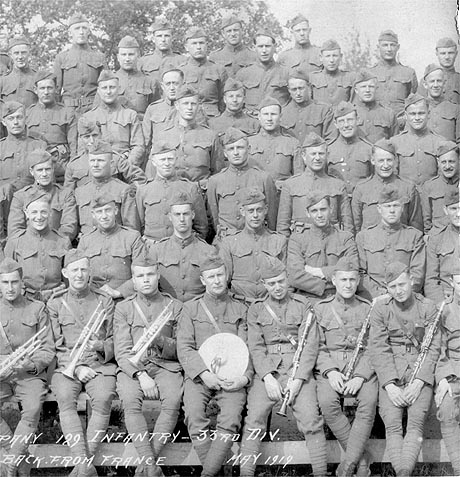<%elseif intNum = 7 then%>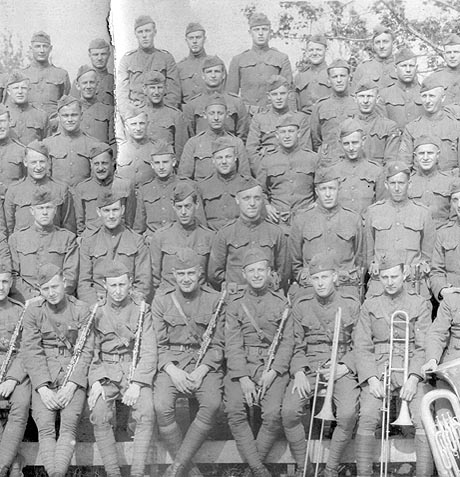<%else%>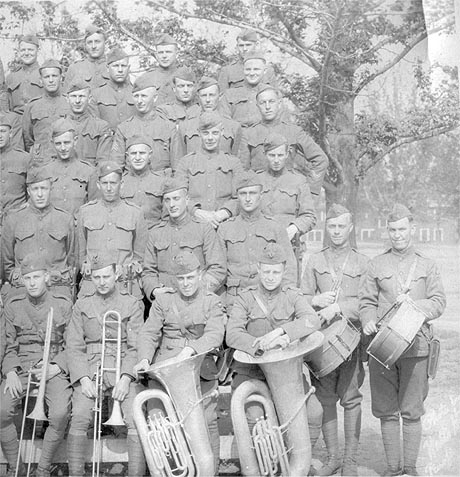<%end if%>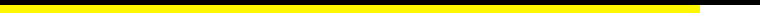Copyright © 2006 James V. GillPage last updatedWe welcome your comments tojimvgill@windstream.net